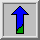# Digital Filters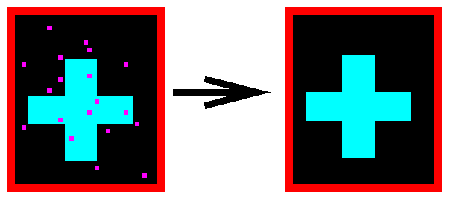# Contents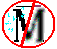Mean Filter - noise reduction NR using mean of neighborhood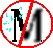Median Filter - NR using median of neighborhood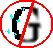Gaussian Smoothing - NR using convolution with a Gaussian smoothing kernel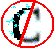Conservative Smoothing - NR using maximum and minimum of neighborhood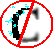Crimmins Speckle Removal - more complex NR operatorFrequency Filters - high and low pass image filters, etcLaplacian/Laplacian of Gaussian Filter - edge detection filter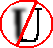Unsharp Filter - edge enhancement filterIn image processing filters are mainly used to suppress either the high frequencies in the image, i.e. smoothing the image, or the low frequencies, i.e. enhancing or detecting edges in the image.

An image can be filtered either in the frequency or in the spatial domain.

The first involves transforming the image into the frequency domain, multiplying it with the frequency filter function and re-transforming the result into the spatial domain. The filter function is shaped so as to attenuate some frequencies and enhance others. For example, a simple lowpass function is 1 for frequencies smaller than the cut-off frequency and 0 for all others.

The corresponding process in the spatial domain is to convolve the input image f(i,j) with the filter function h(i,j). This can be written as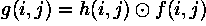The mathematical operation is identical to the multiplication in the frequency space, but the results of the digital implementations vary, since we have to approximate the filter function with a discrete and finite kernel.

The discrete convolution can be defined as a `shift and multiply' operation, where we shift the kernel over the image and multiply its value with the corresponding pixel values of the image. For a square kernel with size M× M, we can calculate the output image with the following formula: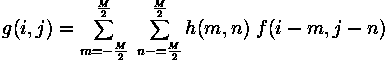Various standard kernels exist for specific applications, where the size and the form of the kernel determine the characteristics of the operation. The most important of them are discussed in this chapter. The kernels for two examples, themean and theLaplacian operator, can be seen in Figure 1.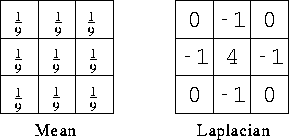Figure 1 Convolution kernel for a mean filter and one form of the discrete Laplacian.

In contrast to the frequency domain, it is possible to implement non-linear filters in the spatial domain. In this case, the summations in the convolution function are replaced with some kind of non-linear operator:For most non-linear filters the elements of h(i,j) are all 1. A commonly used non-linear operator is themedian, which returns the `middle' of the input values.